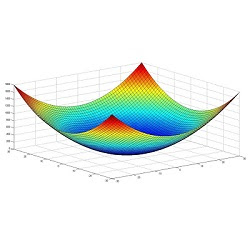# Matlab Alternative Softwares For Linux/Ubuntu/Linux MintFor numerical computation, data analysis, usually Matlab software is used but it is not necessarily best software available. The main thing is that Matlab is commercial and expensive. Students of Machine learning always wondering what other alternative available for them that is powerful and can be free of cost, which means students don't have to spend their money on a proprietary software. I received a request from people who were looking for Matlab alternatives, so I decided to share with all of you, here are some best available alternatives to Matlab for Linux/Ubuntu/Linux Mint. These software are available in Ubuntu universe repository, also you can build from source if needed.

### 1: GNU Octave

GNU Octave is a high-level interpreted language, primarily intended for numerical computations. It provides capabilities for the numerical solution of linear and nonlinear problems, and for performing other numerical experiments. It also provides extensive graphics capabilities for data visualization and manipulation. Octave is normally used through its interactive command line interface, but it can also be used to write non-interactive programs. The Octave language is quite similar to Matlab so that most programs are easily portable.
Octave has extensive tools for solving common numerical linear algebra problems, finding the roots of nonlinear equations, integrating ordinary functions, manipulating polynomials, and integrating ordinary differential and differential-algebraic equations. It is easily extensible and customizable via user-defined functions written in Octave's own language, or using dynamically loaded modules written in C++, C, Fortran, or other languages.

To install GNU Octave in Ubuntu/Linux Mint from Universe repository open Terminal (Press Ctrl+Alt+T) and copy the following commands in the terminal:

### 2: Genius

Genius is a general purpose calculator program similar in some aspects to BC, Matlab, Maple or Mathematica. It is useful both as a simple calculator and as a research or educational tool. The syntax is very intuitive and is designed to mimic how mathematics is usually written. GEL is the name of its extension language, it stands for Genius Extension Language. In fact, many of the standard genius functions are written in GEL itself.
It can do: Arbitrary precision integers, multiple precision floats; Rational numbers, stored as quotient and denominator; Complex numbers, stored in Cartesian coordinates as usual; Math-like-looking expressions, tries to be as much a what-you-mean-is-what-Genius-understands, up to a limit of course; Matrix calculations/Linear Algebra, with many related functions; Number theory; Calculus, numerical and even very limited symbolic calculations; Statistics, all the basic statistical functions; Numerical equation solving, polynomial roots, etc.; Combinatorics; Most common elementary / trigonometric functions; Modular arithmetic, including inversions and modular arithmetic on matrices; A complete programming language, with automatic typing. In fact large part of Genius standard library is written in GEL; 2D Function line plots, standard 2D graphs of up to 10 functions at once, with possibility to export to EPS or PNG; Parametric plots, with possibility to export to EPS or PNG; 3D Function surface plots, with possibility to export to EPS or PNG; Slopefield/Vectorfield plotting.

To install Genius in Ubuntu/Linux Mint from Universe repository open Terminal (Press Ctrl+Alt+T) and copy the following commands in the terminal:

### 3: Sagemath

Sage is a free open-source mathematics software system licensed under the GPL. It combines the power of many existing open-source packages into a common Python-based interface. It's aim to creating a viable free open source alternative to Magma, Maple, Mathematica and Matlab. Sage is built out of nearly 100 open-source packages and features a unified interface. Sage can be used to study elementary and advanced, pure and applied mathematics. This includes a huge range of mathematics, including basic algebra, calculus, elementary to very advanced number theory, cryptography, numerical computation, commutative algebra, group theory, combinatorics, graph theory, exact linear algebra and much more. It combines various software packages and seamlessly integrates their functionality into a common experience.

To install latest Sagemath in Ubuntu 14.04/12.04/Linux Mint 17/13 open Terminal (Press Ctrl+Alt+T) and copy the following commands in the terminal:

To install Sagemath in Ubuntu 14.10 or any Ubuntu/Linux Mint from Universe repository open Terminal (Press Ctrl+Alt+T) and copy the following commands in the terminal:

### 4: Scilab

Scilab is a scientific software for numerical computations which is matrix-based. Scilab contains hundreds of built-in mathematical functions, rich data structures (including polynomials, rationals, linear systems, lists, etc...) and comes with a number of specific toolboxes for control, signal processing, etc. Scilab is free and open source software for numerical computation providing a powerful computing environment for engineering and scientific applications. It also provides Xcos, a graphical editor to design hybrid dynamical systems models. Models can be designed, loaded, saved, compiled and simulated. Stable and efficient solution for industrial and academics needs, Xcos provides functionalities for modeling of mechanical systems (automotive, aeronautics...), hydraulic circuits (dam, pipe modeling...), control systems, etc. Modelica capabilities are also provided.

To install Scilab in Ubuntu/Linux Mint from Universe repository open Terminal (Press Ctrl+Alt+T) and copy the following commands in the terminal: